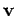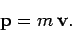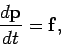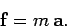Next: Hooke's law Up: Newton's laws of motion Previous: Newton's first law of

## Newton's second law of motion

Newton used the word motion'' to mean what we nowadays call momentum. The momentumof a body is simply defined as the product of its massand its velocity: i.e.,(92)

Newton's second law of motion is summed up in the equation(93)

where the vectorrepresents the net influence, or force, exerted on the object, whose motion is under investigation, by other objects. For the case of a object with constant mass, the above law reduces to its more conventional form(94)

In other words, the net force exerted on a given object by other objects equals the product of that object's mass and its acceleration. Of course, this law is entirely devoid of content unless we have some independent means of quantifying the forces exerted between different objects.Next: Hooke's law Up: Newton's laws of motion Previous: Newton's first law of
Richard Fitzpatrick 2006-02-02